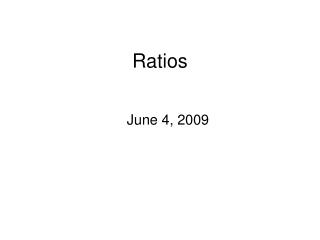DownloadDownload PresentationRatios

# Ratios

Télécharger la présentation## Ratios

- - - - - - - - - - - - - - - - - - - - - - - - - - - E N D - - - - - - - - - - - - - - - - - - - - - - - - - - -
##### Presentation Transcript

1. Ratios June 4, 2009

2. Euler Product

3. To get the ratios conjecture • Follow the recipe for moments • Replace the numerator L’s by apprx fnc eq • Replace the denominator L’s by their full Dirichlet series • Multiply out • Bring the average over the family inside • Replace averages by their expected values using the appropriate harmonics of the family • Extend all coefficient sums, extract zeta’s

4. Application to Mollifying

5. Use Perron’s formula

6. RATIOS THEOREM (UNITARY)

7. RATIOS THEOREM (ORTHOGONAL)

8. RATIOS THEOREM (SYMPLECTIC)

9. Ratios conjecture (zeta)

10. Ratios conjecture (zeta)

11. Application to pair correlation

12. Montgomery, 1971 – pair correlation

13. Montgomery’s Pair Correlation Conjecture

14. Picture by A. Odlyzko 79 million zeros around the th zero

15. First 100000 zeros

16. zeros around the th zero

17. Bogomolny and Keating

18. Refined pair-correlation conjecture (Bogomolny-Keating, Conrey-Snaith)

19. The ratios approach to lower order terms

20. We want to evaluate T 1-a 1/2 a

21. Move contours to the right, becomes

22. Difference between theory and numerics:

23. For large T:

24. Hejhal, 1994 - triple correlation where the Fourier transform of f has support on the hexagon with vertices (1,0),(0,1),(-1,1),(-1,0),(0,-1),(1,-1), and

25. Rudnick and Sarnak, 1996 n-correlation: Bogomolny and Keating, 1995,1996 Heuristic using Hardy-Littlewood conjecture to obtain large T scaling limit Scaling limit for the n-point correlation function, again with restricted support of the Fourier transform of the test function.

26. Triple correlation using ratios:

27. A,B,Q,P are expressions involving primes (see Bogomolny, Keating, Phys.Rev.Lett.,1996)

28. Applications to lower order terms in one-level densities

29. One-level density

30. Application to discrete moments

31. Steve Gonek proved this, assuming RH, for k=1. The RMT analogue of the conjecture is a theorem due to Chris Hughes.